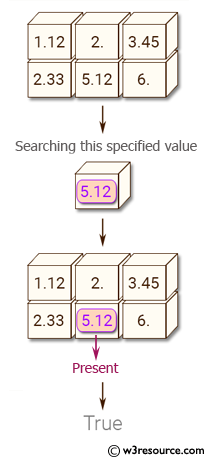﻿ NumPy: Test whether specified values are present in an array - w3resource# NumPy: Test whether specified values are present in an array

## NumPy: Array Object Exercise-65 with Solution

Write a NumPy program to test whether specified values are present in an array.

Pictorial Presentation:Sample Solution:-

Python Code:

``````import numpy as np
x = np.array([[1.12, 2.0, 3.45], [2.33, 5.12, 6.0]], float)
print("Original array:")
print(x)
print(2 in x)
print(0 in x)
print(6 in x)
print(2.3 in x)
print(5.12 in x)
```
```

Sample Output:

```Original array:
[[ 1.12  2.    3.45]
[ 2.33  5.12  6.  ]]
True
False
True
False
True
```

Python Code Editor:

Have another way to solve this solution? Contribute your code (and comments) through Disqus.

What is the difficulty level of this exercise?

Test your Python skills with w3resource's quiz

﻿

## Python: Tips of the Day

Set comprehension:

```>>> m = {x ** 2 for x in range(5)}
>>> m
{0, 1, 4, 9, 16}
```